### Home > CALC > Chapter 6 > Lesson 6.3.3 > Problem6-104

6-104.
1. A calculator company found that the cost of producing x graphing calculators per day is C(x) = 5x + e0.02x . (This ignores the original research and development cost, which is quite large.) Homework Help ✎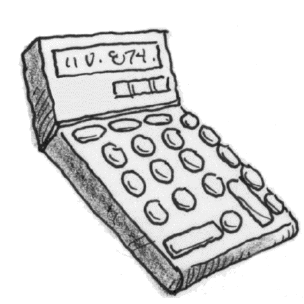1. If each calculator is priced at $90, find a daily revenue function, R(x), which calculates the income for x calculators sold. 2. Find the profit function, P(x), which calculates the profit per day when x calculators are produced and each later sold for$90.

3. Find the daily production that will maximize the profit.

4. What is the maximum daily profit?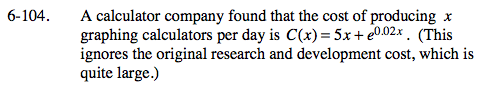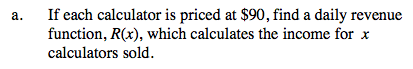R(x) = 90x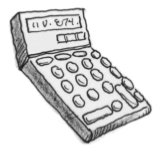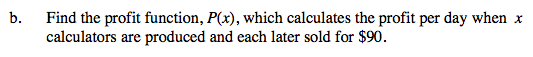Profit is the difference between revenue and cost of product.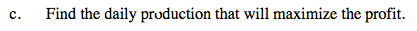Optimize your profit function. We are looking for an x-value.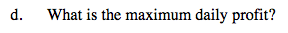Find the P(x) value that corresponds to your answer in part (c).